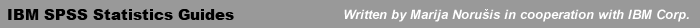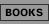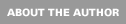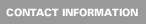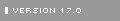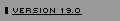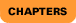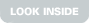IBM SPSS Statistics 19 Advanced Statistical Procedures Companion: Chapters

 Model Selection in Loglinear Analysis. Model formulation; parameters in saturated models; hypothesis testing; convergence; goodness-of-fit tests; hierarchical models; generating classes; model selection with backward elimination. Logit Loglinear Analysis. Dichotomous logit model; loglinear representation; parameter estimates; goodness-of-fit statistics; measures of dispersion and association; polychotomous logit model; interpreting parameters; examining residuals; introducing covariates. Multinomial Logistic Regression. Baseline logits; likelihood-ratio tests for models and individual effects; evaluating the model; calculating predicted probabilities; the classification table; goodness-of-fit tests; residuals; pseudo R-square measures; overdispersion; model selection; matched case-control studies. Ordinal Regression. Modeling cumulative counts; parameter estimates; testing for parallel lines; model fit; observed and expected counts; measures of strength of association; classifying cases; link functions; fitting a heteroscedastic probit model; fitting location and scale parameters. Probit Regression. Probit and logit response models; confidence intervals for effective dosages; comparing groups; comparing relative potencies; estimating the natural response rate; multiple stimuli. Kaplan-Meier Survival Analysis. Calculating survival time; estimating the survival function, the conditional probability of survival, and the cumulative probability of survival; plotting survival functions; comparing survival functions; stratified comparisons. Life Tables. Calculating survival probabilities; assumptions; observations lost to follow-up; plotting survival functions; comparing survival functions. Cox Regression. The model; proportional hazards assumption; coding categorical variables; interpreting the regression coefficients; baseline hazard and cumulative survival rates; global tests of the model; checking the proportional hazards assumption; stratification; log-minus-log survival plot; identifying influential cases; examining residuals; partial (Schoenfeld) residuals; martingale residuals; variable-selection methods; time-dependent covariates; specifying a time-dependent covariate; calculating segmented time-dependent covariates; testing the proportional hazards assumption with a time-dependent covariate; fitting a conditional logistic regression model. Variance Components. Factors, effects, and models; model for one-way classification; estimation methods; negative variance estimates; nested design model for two-way classification; univariate repeated measures analysis; using a Mixed Models Approach; distribution assumptions; estimation methods. Linear Mixed Models. Background; Unconditional random-effects models; hierarchical models; random-coefficient model; model with school-level and individual-level covariates; three-level hierarchical model; repeated measurements; selecting a residual covariance structure. Generalized Linear Models. Background; selecting the type of model; selecting a link function; fitting a logistic regression; testing hypotheses about coefficients; fitting a Poisson rate model; fitting a probit model; fitting a Poisson count model; fitting a loglinear model; fitting a gamma distribution to survival time. Generalized Estimation Equations. The GEE model; data organization; specifying the model; tests of model effects; correlation structure; comparing correlation structures; comparing models; variance estimators; fitting a Poisson model. Generalized Linear Mixed Models. Using the GLMM procedure; describing data structure; identifying field and effects; examining results in the model viewer; logistic regression with correlated data; analyzing repeated measures data; fitting a Poisson model. Nonlinear Regression. The nonlinear model; transforming nonlinear models; intrinsically nonlinear models; fitting a logistic population growth model; finding starting values; approximate confidence intervals for the parameters;bootstrapped estimates; starting values from previous analysis; linear approximation; computational issues; common models for nonlinear regression; specifying a segmented model. Two-Stage Least-Squares Regression. Demand-price-income economic model; estimation with ordinary least squares; feedback and correlated errors; estimation with two-stage least squares. Weighted Least-Squares Regression. Diagnosing the problem; estimating weights; examining the log-likelihood function; the WLS solution; estimating weights from replicates; diagnostics from the linear regression procedure. Multidimensional Scaling. Data, models, and multidimensional scaling analysis; nature of data analyzed in MDS; measurement level of data; shape of data; conditionality of data; missing data; multivariate data; classical MDS; Euclidean model; details of CMDS; Replicated MDS; Weighted MDS; geometry of the weighted Euclidean model; algebra of the weighted Euclidean model; matrix algebra of the weighted Euclidean model; Weirdness index; flattened weights.© 2005-2011 Marija Norušis: IBM SPSS Statistics Guides Site designed by Kenny Kim Photography